## Section29.8Coulomb's Law Bootcamp

### Subsection29.8.5Miscellaneous

A wire of length $L$ carries a uniform charge $\lambda$ (units: $\text{C/m}$). Find electric field at points (a) $\text{P}_1$ and (b) $\text{P}_2\text{.}$Hint

(a) Use $x$ axis along the wire and find field of an infinitesimal element of the wire. Integrate to get the field. (b) Avoid the field point on the wire by working out field at a point infinitesimally left and at a point infinitesimally to the right.

(a) $k\;\frac{\lambda L}{a(L+a)}\text{,}$ (b) $k\lambda \left[ \frac{2b - L}{b(L-b)} \right]\text{.}$

Solution 1 (a)

(a) Let us place $x$ axis along the wire with origin at one end as shown in Figure 29.8.29. Each charge element on the wire will have only $x$ component of the electric field. For instance, electric field of the element $dq = \lambda\; dx$ will be

\begin{equation*} dE_x = k \frac{\lambda\; dx}{ \left( L+a-x \right)^2}. \end{equation*}

Integrating this from $x=0$ to $x=L$ will give the net field at $\text{P}_1\text{.}$

\begin{align*} E_x \amp= k\lambda\; \int_0^L \frac{dx}{ \left( L+a-x \right)^2} \\ \amp=- k\lambda\; \int_{L+a}^a \frac{dy}{ y^2} \ \ \ (y=L+a-x) \\ \amp= k\lambda\; \left( \frac{1}{a} - \frac{1}{L+a}\right) = k\;\frac{\lambda L}{a(L+a)}. \end{align*}

Note that this is not $kq/r^2\text{.}$ If we write $q=\lambda L$ for net charge on the wire and place the entire charge at the center, the field at $\text{P}_1$ will be

\begin{equation*} E = k \frac{\lambda L}{ (\frac{L}{2} + a)^2 }. \end{equation*}

This is definitely very different from the answer we found for the problem. Only in the limit $a \gg L \text{,}$ the two expressions become in indistinguishable.

Solution 2 (b)

(b) Here we see that electric field of charge on points to the left of $\text{P}_2$ is pointed to right but points on the right are pointed to left. Using (a), we can write the electric field of left and right separately. But, when we do this calculation naively, by setting $a=0$ in the answer for (a), you will run into trouble of dividing by zero. To avoid that, we find field at an infintesimal left of $\text{P}_2$ and infinitesimal right of $\text{P}_2\text{.}$

Let $\epsilon$ denote an infinitesimal length. then, field from the left of $\text{P}_2$ at a point infintesimal left of $\text{P}_2$ will be

\begin{equation*} E_{\text{left},x} = k\lambda\; \left( \frac{1}{\epsilon} - \frac{1}{L}\right) \end{equation*}

and at a point infintesimal right of $\text{P}_2$

\begin{equation*} E_{\text{right},x} = -k\lambda\; \left( \frac{1}{\epsilon} - \frac{1}{L-b}\right) \end{equation*}

Adding them, we find that the arbitrarily chosen infinitesimal size $\epsilon$ cancels out giving us the following, which correctly gives zero for the midpoint.

\begin{align*} E_x \amp = E_{\text{left},x} + E_{\text{right},x} = k\lambda \left[ \frac{2b - L}{b(L-b)} \right] \end{align*}

A non-conducting wire shaped in a half-circle arc of radius $R$ has a uniform charge $\lambda\text{.}$ Find the electric field at the center.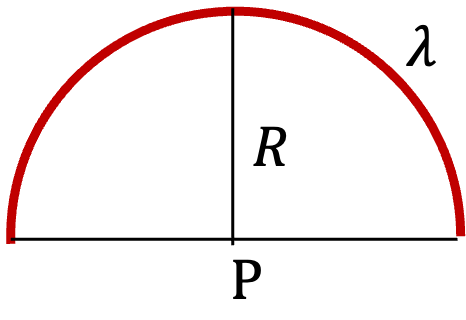Hint

Place the arc symmetrically about $y$ axis. Work out $y$ field of an element of arc and then integrate.

Magnitude $\frac{2 k \lambda}{R}$ in the direction down if charge positive.

Solution

Let us place the arc symmetrically as shown here. From symmetry about $y$-axis, net electric field from all elements of the arc will have only $y$ component nonzero.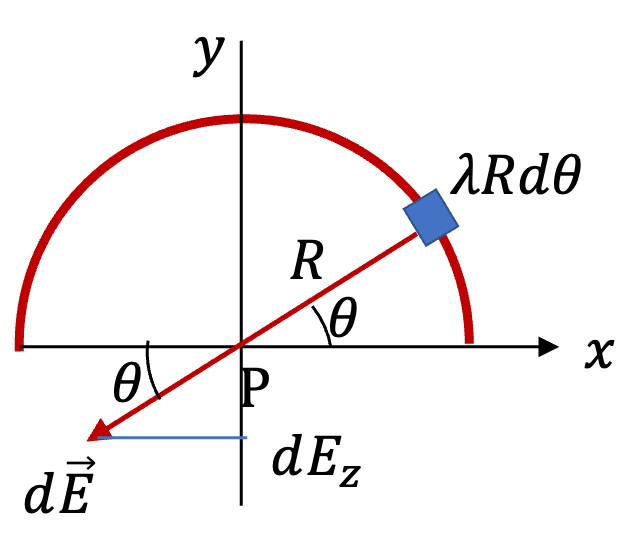Therefore, we focus on obtaining $y$ component of one representative element. Magnitude of $d\vec E$ from element of size $Rd\theta$ is relatively easy to see.

\begin{equation*} dE = k \frac{dq}{R^2} = k \frac{\lambda R d\theta}{R^2}. \end{equation*}

Now, we want $y$ component of this. From the figure, we see that it is just multiplied by $\sin\theta$ and $-1$ since projection is on negative $y$ axis.

\begin{equation*} dE_z = - k \frac{\lambda R d\theta}{R^2}\ \sin\theta. \end{equation*}

We integrate it from $\theta=0$ to $\theta=\pi$ to get the net field.

\begin{align*} E_z \amp = - \frac{k \lambda}{R} \int_0^\pi \sin\theta\: d\theta = -\frac{2 k \lambda}{R}. \end{align*}

Therefore, magnitude is $\frac{2 k |\lambda|}{R}$ and direction is towards negative $y$ axis if charge positive.

A square of side $a$ is charged with uniform charge density of $\lambda$ (units: $\text{C/m}$).

(a) Find electric field at a distance $h$ above the center of the square.(b) Show that in the limit $h \gt \gt a\text{,}$ the formula becomes that of a point charge at the center with charge equal to total charge on the square.

Strategy:

You may make use of the the electric field of a straight wire of length $L$ with charge density $\lambda$ that has the following formula for electric field at a distanc $D$ away from its center.

\begin{equation*} \vec E_\text{straight} = \hat u\: k \frac{2\lambda L}{ D\sqrt{L^2 + 4D^2}}, \end{equation*}

where unit vector $\hat u$ is pointed away from the wire.

Hint

Only vertical component will survive. Find vertical component of the field by one side and multiply by four.

(a) $E_z = \frac{ 4k\lambda a h }{(h^2 + a^2/4)\sqrt{4h^2 + 2a^2}}\text{.}$

Solution 1 (a)

(a) Electric field of each side will be in a different direction. But, from symmetry, it is clear that horizontal components of the field from opposite sides of the square will cancel out. Therefore, we just need o work out the vertical component of one wire and multiply by four.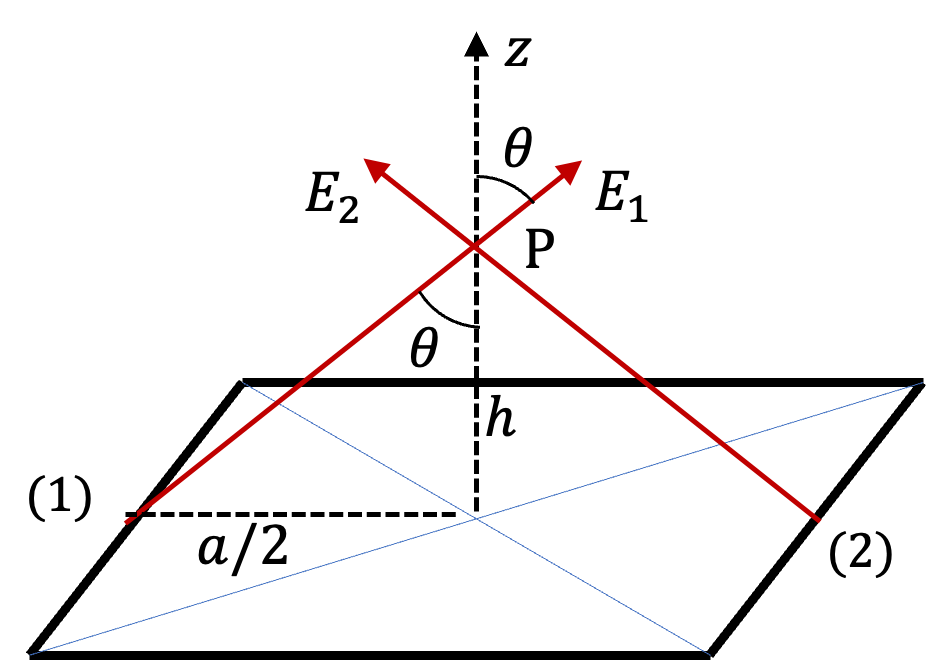Vertical component will be along $z$ axis in the figure. So, we will just call it as $E_z\text{.}$ Now, the distance of P from wire (1) in figure is

\begin{equation*} D = \sqrt{h^2 + (a/2)^2}. \end{equation*}

The $z$ component will be related to the amplitude by $\cos\theta\text{.}$

\begin{equation*} E_{1z} = E_1\:\cos\theta = E_1\:\frac{h}{D}. \end{equation*}

Therefore, with $L=a$ in the formula for magnitude of field from one wire, we can get net electric field at P to be

\begin{align*} E_z \amp = 4\times E_{1z} = 4\times E_1\times \frac{h}{D} \\ \amp = 4\times k \frac{2\lambda a}{ D\sqrt{a^2 + 4D^2}} \times \frac{h}{D} = \frac{ 8k\lambda a h }{D^2\sqrt{a^2 + 4D^2}}. \end{align*}

We can replace $D$ with $\sqrt{h^2 + (a/2)^2}$ and simplify to

\begin{equation*} E_z = \frac{ 8k\lambda a h }{(h^2 + a^2/4)\sqrt{4h^2 + 2a^2}}. \end{equation*}
Solution 2 (b)

When we take limit $a/h \rightarrow 0\text{,}$ we will get the following in the leading order.

\begin{equation*} E_z = \frac{ 8k\lambda a h }{2h^3} = k\frac{ q }{h^2}, \end{equation*}

where $q=4a\lambda\text{,}$ the total charge on the square. This is the field of a point charge $q$ placed at the center.

Suppose charges are painted on two large nonconducting sheets so that they have equal charge densities of opposite types, $\pm \sigma\text{.}$ Then, the two sheets are placed perpendicularly to each other.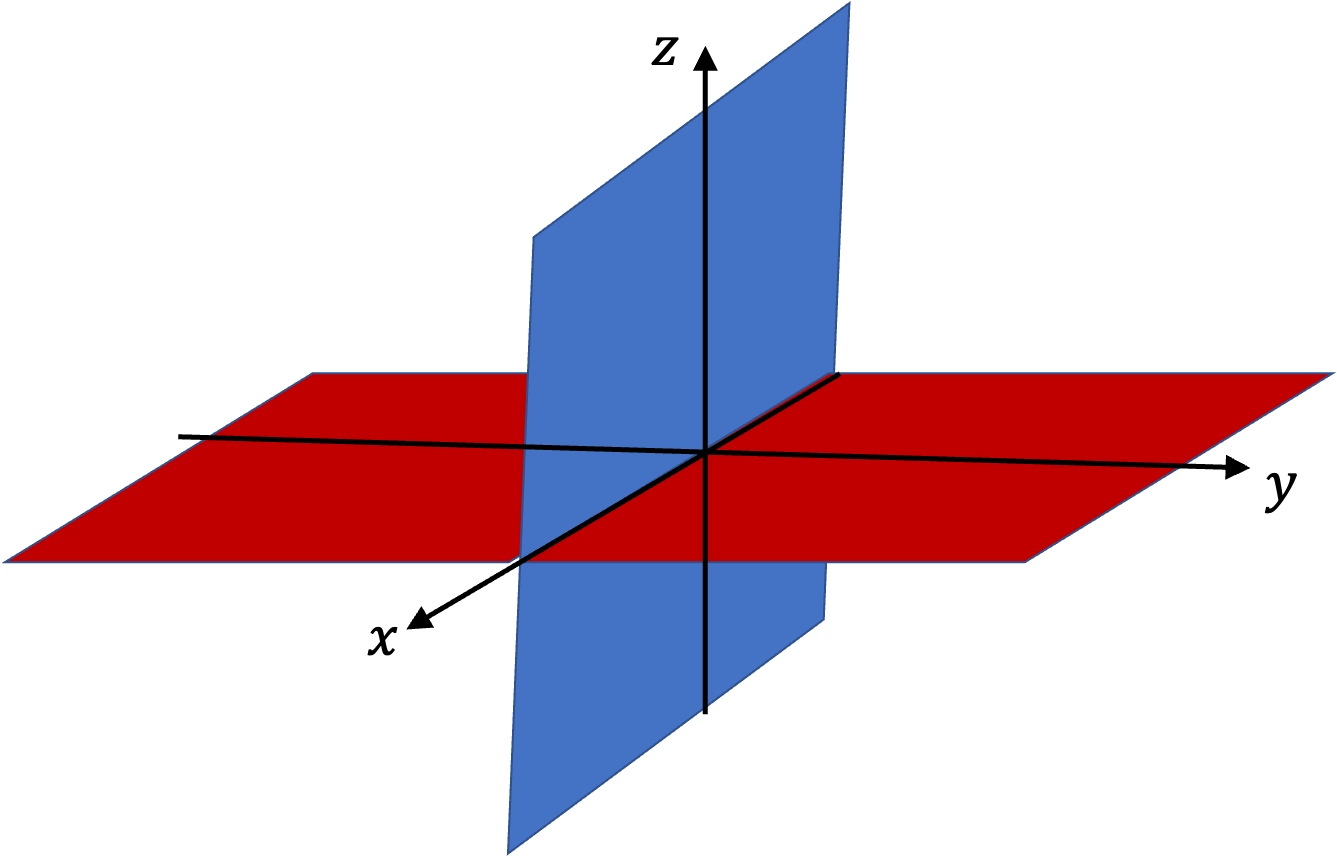You may think of positive sheet being $xy$ plane and the negative sheet as $xz$ plane. Find electric field everywhere.

Hint

Use superposition of field of each of the two sheets.

Magnitude $\sqrt{2}\frac{\sigma}{\epsilon_0}$ and directions vary in $yz$ plane with counterclockwise angles from positive $y$ axis: $135^\circ, y \gt 0, z \gt 0;$ $225^\circ, y \gt 0, z \lt 0;$ $45^\circ, y \lt 0, z \gt 0;$ $315^\circ, y \lt 0,z \lt 0.$

Solution

First notice that electric field of each sheet is independently known to be

\begin{align*} E_{z}^{(+)} \amp = \begin{cases} \frac{\sigma}{\epsilon_0} \amp z \gt 0,\\ -\frac{\sigma}{\epsilon_0} \amp z \lt 0, \end{cases}\\ E_{y}^{(-)} \amp = \begin{cases} -\frac{\sigma}{\epsilon_0} \amp y \gt 0,\\ +\frac{\sigma}{\epsilon_0} \amp y \lt 0. \end{cases} \end{align*}

The other components are all zero. By superposition principle, we know that the net electric field at any point will be a vector sum of these two. There are four regions based on the $y$ and $z$ values.

\begin{equation*} \vec E = \begin{cases} -\frac{\sigma}{\epsilon_0}\hat u_y + \frac{\sigma}{\epsilon_0}\hat u_z \amp y \gt 0, z \gt 0,\\ -\frac{\sigma}{\epsilon_0}\hat u_y - \frac{\sigma}{\epsilon_0}\hat u_z \amp y \gt 0, z \lt 0,\\ \frac{\sigma}{\epsilon_0}\hat u_y + \frac{\sigma}{\epsilon_0}\hat u_z \amp y \lt 0, z \gt 0,\\ \frac{\sigma}{\epsilon_0}\hat u_y - \frac{\sigma}{\epsilon_0}\hat u_z \amp y \lt 0, z \lt 0. \end{cases} \end{equation*}

From these we get the magnitude to be same everywhere, $E=\sqrt{2}\frac{\sigma}{\epsilon_0}\text{,}$ and direction being different for different cases. Let $\theta$ be counterclockwise angle with respect to positive $y$ axis in $yz$ plane.

\begin{equation*} \theta = \begin{cases} 135^\circ \amp y \gt 0, z \gt 0,\\ 225^\circ \amp y \gt 0, z \lt 0,\\ 45^\circ \amp y \lt 0, z \gt 0,\\ 315^\circ \amp y \lt 0,z \lt 0. \end{cases} \end{equation*}

A hollow drum of radius $R$ and height $h$ is charged with uniform charge density of $\sigma$ (units: $\text{C/m}^2$). Consider a point P on the axis of the cylinder. (a) Find electric field at this point. For ease of calculation, place the point outside the cylinder. (b) Show that the electric field can also be written as $\frac{\sigma}{2\epsilon_0} (\sin\theta_1 - \sin\theta_2).$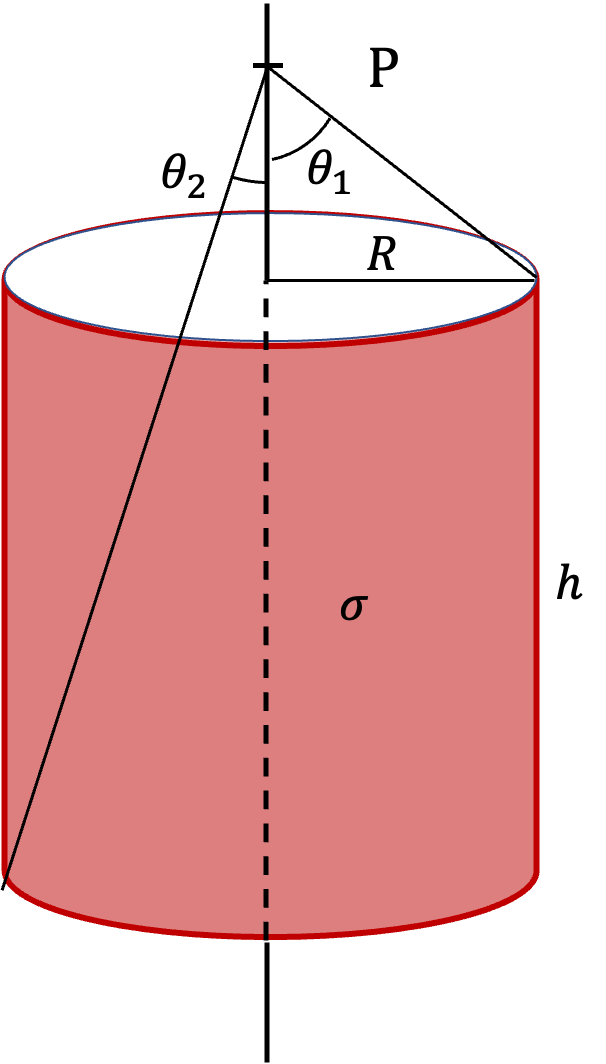Hint

Use ring-shaped element and the formula for electric field of the ring to set up $dE_z\text{.}$

(a) $E_z = 2\pi k \sigma \left[ \frac{R}{\sqrt{R^2 + (z_0-h)^2}} - \frac{R}{\sqrt{R^2 + z_0^2}} \right]\text{.}$

Solution 1 (a)

(a) We will use a ring-shaped element of the drum between $z$ and $z+dz\text{.}$ The area of this strip is $2\pi R dz\text{.}$ Therefore, amount of charge in this ring is $dq = \sigma \times (2\pi R dz)\text{.}$ Since, point P is a distance $z_0 - z$ from this ring, we can immediately write the formla for the $z$ component of electric field at P using the formula for a ring.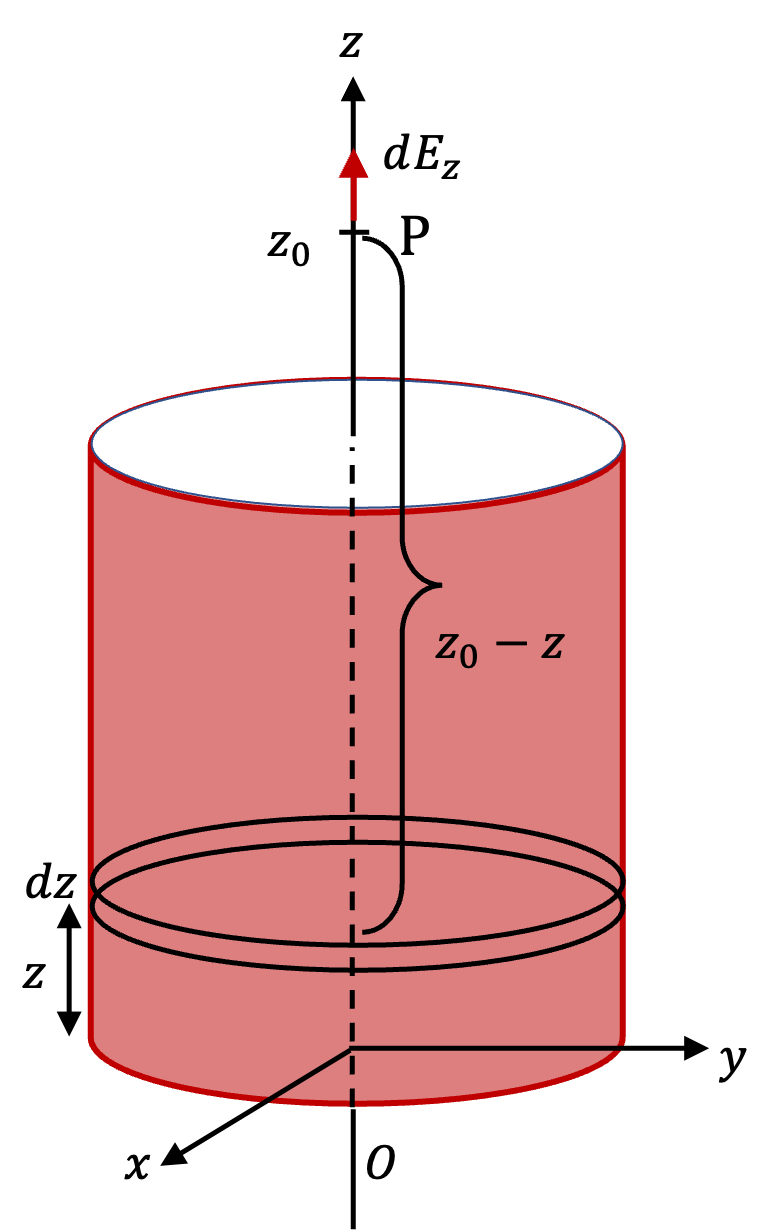\begin{align*} dE_z \amp = k\frac{D\; dq}{ \left( R^2 + D^2 \right) }\\ \amp = 2\pi k R \sigma \frac{(z_0-z)\; dz}{ \left( R^2 + (z_0-z)^2 \right) }. \end{align*}

Integrating this from $z=0$ to $z=h$ gives the net electric field at P. Integration is helped by successive change of variables: (1) change to $a=z_0-z\text{,}$ (2) then to $b=a^2\text{,}$ and (3) then $c=R^2 + b\text{.}$ The final result you should get is

\begin{equation*} E_z = 2\pi k \sigma \left[ \frac{R}{\sqrt{R^2 + (z_0-h)^2}} - \frac{R}{\sqrt{R^2 + z_0^2}} \right]. \end{equation*}
Solution 2 (b)

(b) From the definition of angles $\theta_1$ and $\theta_2\text{,}$ it is clear that

\begin{align*} \amp \sin\theta_1 = \frac{R}{\sqrt{R^2 + (z_0-h)^2}}, \\ \amp \sin\theta_2 = \frac{R}{\sqrt{R^2 + z_0^2}}. \end{align*}

We also know that $k = 1/4\pi\epsilon_0\text{.}$ Therefore, we get

\begin{equation*} E_z = \frac{\sigma}{2\epsilon_0}\left( \sin\theta_1 - \sin\theta_2\right). \end{equation*}